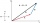# The fence

I'm building a fence. Late is rounded up in semicircle. The tops of late in the field between the columns are to copy an imaginary circle. The tip of the first and last lath in the field is a circle whose radius is unknown. The length of the circle chord is 180cm. The height of the arc in the middle of the chord is 23cm. There are 16 lates and their axes are 12 cm apart. Please calculate the height of lates no.2-8. i.e. half of circle arc.

Result

r =  187.6 cm
y2 =  170.6 cm
y3 =  175.6 cm
y4 =  179.6 cm
y5 =  182.8 cm
y6 =  185.2 cm
y7 =  186.7 cm
y8 =  187.5 cm

#### Solution:Leave us a comment of example and its solution (i.e. if it is still somewhat unclear...):

Showing 0 comments:Be the first to comment!#### To solve this example are needed these knowledge from mathematics:

For Basic calculations in analytic geometry is helpful line slope calculator. From coordinates of two points in the plane it calculate slope, normal and parametric line equation(s), slope, directional angle, direction vector, the length of segment, intersections the coordinate axes etc. Pythagorean theorem is the base for the right triangle calculator.

## Next similar examples:

1. DistanceWha is the distance between the origin and the point (18; 22)?
2. Theorem proveWe want to prove the sentense: If the natural number n is divisible by six, then n is divisible by three. From what assumption we started?
3. Triangle IRTIn isosceles right triangle ABC with right angle at vertex C is coordinates: A (-1, 2); C (-5, -2) Calculate the length of segment AB.
4. CenterCalculate the coordinates of the circle center: ?
5. Chord - TS v2The radius of circle k measures 87 cm. Chord GH = 22 cm. What is TS?
6. Equation of circlefind an equation of the circle with indicated properties: a. center at (-3,5), diameter 20. b. center at origin and diameter 16.
7. Sphere cutsAt what distance from the center intersects sphere with radius R = 56 plane, if the cut area and area of the main sphere circle is in ratio 1/2.
8. Circle - AGFind the coordinates of circle and its diameter if its equation is: ?
9. Circle annulusThere are 2 concentric circles in the figure. Chord of larger circle 10 cm long is tangent to the smaller circle. What are does annulus have?
10. Common chordTwo circles with radius 17 cm and 20 cm are intersect at two points. Its common chord is long 27 cm. What is the distance of the centers of these circles?
11. RT and circlesSolve right triangle if the radius of inscribed circle is r=9 and radius of circumscribed circle is R=23.
12. CandiesIn the box are 12 candies that look the same. Three of them are filled with nougat, five by nuts, four by cream. At least how many candies must Ivan choose to satisfy itself that the selection of two with the same filling? ?
13. TetrahedronCalculate height and volume of a regular tetrahedron whose edge has a length 18 cm.
14. ABS CNCalculate the absolute value of complex number -15-29i.
15. Euclid2In right triangle ABC with right angle at C is given side a=27 and height v=12. Calculate the perimeter of the triangle.
16. Coordinates of vectorDetermine the coordinate of a vector u=CD if C(19;-7) and D(-16;-5)
17. Vector - basic operationsThere are given points A [-9; -2] B [2; 16] C [16; -2] and D [12; 18] a. Determine the coordinates of the vectors u=AB v=CD s=DB b. Calculate the sum of the vectors u + v c. Calculate difference of vectors u-v d. Determine the coordinates of the vector w.